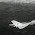## Tuesday, September 28, 2010

### A "Glass Slab" Model

Here is the second tutorial as provided by Barton Paul Levenson. Again, I'll present the tutorial, followed by an explanation of the code added to the github repository. I'll continue to use these simple models to demonstrate some of the key concepts we'll be using to build GoGCM (as I did with the sharing post).

Here's the tutorial.

A "Glass Slab" Model
Written by Barton Paul Levenson

Now we add an atmosphere to create a "glass slab" model. Model 1 just had two factors affecting the Earth's surface temperature: sunlight and reflection. Model 2 adds IR back-radiation.

The Earth's albedo is assumed to apply to the very top of the atmosphere, giving F = 237 W m-2 from the 342 W m-2 which actually comes from the sun.

The surface absorbs all sunlight and all IR that falls on it. The atmosphere absorbs all IR, but no sunlight. Energy balances for atmosphere and surface, respectively, are:

Atmosphere: \begin{equation}F_s=2F_a\end{equation} Surface: \begin{equation}F+F_a=F_s\end{equation}
Here, F is the incoming sunlight, as defined in model 1 from the Earth's albedo and solar constant. Fa is the amount radiated from the atmosphere--since it has both a top and a bottom, it radiates Fa in each direction. Fs is the amount radiated from the Earth, which only goes up. The atmosphere has Fs from the ground as its only energy input; its output is 2 Fa. The Earth gets both F from the sun and Fa from the atmosphere; its output is Fs.

Assuming perfect emissivity, we can substitute in the Stefan-Boltzmann law to rephrase these equations in terms of temperature:

\begin{equation}\sigma T_s^4=2\sigma T_a^4\end{equation} \begin{equation}F+\sigma T_a^4=\sigma T_s^4\end{equation}
Dividing through all terms by σ to simplify, we get

\begin{equation}T_s^4=2T_a^4\end{equation} \begin{equation}\frac{F}{\sigma}+T_a^4=T_s^4\end{equation}
Ts4 occurs on the left-hand side (LHS) of the top equation and the right-hand side (RHS) of the second. We can therefore eliminate Ts between the two equations and find that

\begin{equation}T_{a}=\left(\frac{F}{\sigma}\right)^{0.25}\end{equation}
And, with more algebra:

\begin{equation}T_{s}=2^{0.25}T_a\end{equation}
yielding Ta = 254 K. Do you recognize the Earth's radiative equilibrium temperature, Te? And Ts = 303 K. The latter figure is 15 K over the actual figure--5% too high--but that's closer than last time.

But Earth's atmosphere isn't equivalent to one blackbody layer in IR-absorbing ability. It's closer to two. We would then have, with layer 1 on top and layer 2 on bottom, and the ground underneath both, T1 = 254 K, T2 = 303 K, and Ts = 335 K, which is too high by 47 K, or 16%. This is worse than model 1! Clearly we're leaving out something important.

Go Code
Arrow

I've added the code to the github repository, under the examples directory as model 002. I've reused the code from model 001, including the improvements as described in the sharing post. I'll keep working on model 002. Let me know if you'd like to see anything in particular.

1.Would it be possible to use mathjax (http://www.mathjax.org/) for typing the math in your posts? It would make them much more readables...

2.Thanks for the link. I'll try looking into this. After a quick look, it seemed like I needed a server to run the code (which I don't have, running this blog on blogger). I saw an example using the mathjax server, however I'll only be able to try this later. I'll let you know how it goes.

3.I've edited the equations above. Thanks for the suggestion. I still haven't figured out how to do equations inline, but for now that should do.

4.Francesco PaparellaOctober 3, 2010 at 11:58 AM

Math looks nice, now, isn't it? :)

5.Yes! Thanks for the tip.

6.Ref: Doubling of the atmospheric layer.

When you double the layers you must take into account the optical depth of each layer. If one layer absorbs 99% of the earth's emitted radiation, then two layers each absorbing 90% of incoming radiation have the same global property. (90% + 10%*90% = 99%)

As you refine the layers, the absorbed amount per layer is lower and lower and the overall temperature trend through the atmosphere stabilizes.

Will

7.Hi Willem, Thanks for the comment.

I agree that the optical depth is important, although this model is simpler than that for now. This model is making the assumption that all solar radiation is being absorbed by the surface, while all emitted radiation is absorbed by the next layer (ie emitted radiation is all IR and all absorbed in the atmosphere).